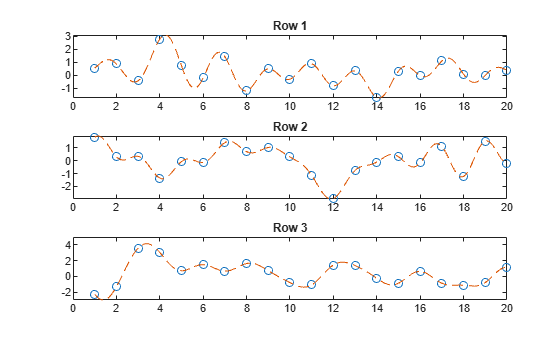interpft

1-D interpolation (FFT method)

Description

example

y = interpft(X,n) interpolates the Fourier transform of the function values in X to produce n equally spaced points. interpft operates on the first dimension whose size does not equal 1.

example

y = interpft(X,n,dim) operates along dimension dim. For example, if X is a matrix, then interpft(X,n,2) operates on the rows of X.

Examples

collapse all

Interpolate 1-D data using the FFT method and visualize the result.

Generate some sample points in the interval $\left[0,3\pi \right]$ for the function $f\left(x\right)={\mathrm{sin}}^{2}\left(x\right)\mathrm{cos}\left(x\right)$. Use a spacing interval dx to ensure the data is evenly spaced. Plot the sample points.

dx = 3*pi/30;
x = 0:dx:3*pi;
f = sin(x).^2 .* cos(x);
plot(x,f,'o')Use FFT interpolation to find the function value at 200 query points.

N = 200;
y = interpft(f,N);

Calculate the spacing of the interpolated data from the spacing of the sample points with dy = dx*length(x)/N, where N is the number of interpolation points. Truncate the data in y to match the sampling density of x2.

dy = dx*length(x)/N;
x2 = 0:dy:3*pi;
y = y(1:length(x2));

Plot the results.

hold on
plot(x2,y,'.')
title('FFT Interpolation of Periodic Function')Generate three separate data sets of normally distributed random numbers. Assume the data is sampled at the positive integers, 1:N. Store the data sets as rows in a matrix.

A = randn(3,20);
x = 1:20;

Interpolate the rows of the matrix at 500 query points each. Specify dim = 2 so that interpft works on the rows of A.

N = 500;
y = interpft(A,N,2);

Calculate the spacing interval of the interpolated data dy. Truncate the data in y to match the sampling density of x2.

dy = length(x)/N;
x2 = 1:dy:20;
y = y(:,1:length(x2));

Plot the results.

subplot(3,1,1)
plot(x,A(1,:)','o');
hold on
plot(x2,y(1,:)','--')
title('Row 1')

subplot(3,1,2)
plot(x,A(2,:)','o');
hold on
plot(x2,y(2,:)','--')
title('Row 2')

subplot(3,1,3)
plot(x,A(3,:)','o');
hold on
plot(x2,y(3,:)','--')
title('Row 3')Input Arguments

collapse all

Input array, specified as a vector, matrix, or multidimensional array. The data in X is assumed to be sampled at an evenly spaced interval of the independent variable. interpft works best with periodic data.

Data Types: single | double
Complex Number Support: Yes

Number of points, specified as a positive integer scalar.

Data Types: single | double

Dimension to operate along, specified as a positive integer scalar. If no value is specified, then the default is the first array dimension whose size does not equal 1.

• interpft(X,n,1) interpolates the columns of X.• interpft(X,n,2) interpolates the rows of X.Example: interpft(X,n,3)

Output Arguments

collapse all

Interpolated points, returned as a vector, matrix, or multidimensional array. If length(X,dim) = m, and X has a sampling interval of dx, then the new sampling interval for y is dy = dx*m/n, where n > m.

If dim is specified, then interpft pads or truncates X to length n in dimension dim, so that size(y,dim) = n.

Algorithms

The interpft function uses the FFT method. The original vector x is transformed to the Fourier domain using fft, and then it is transformed back with more points.